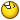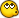Calculating tank's litres

gaboon

How do you calculate this?

gaboon

Seek and thee shall findThanks Alfie

Hill

It is very easy. Take a tank of 2000mm x 500mm x 500mm for example.

Divide measurement by 100 to get into decimeters. Then multiply. 20x5x5 equals 500 litres. Quicker than looking up a webpage.Reef Maniac

... Divide measurement by 100 to get into decimeters.
Wow, it's been a while since I've heard that measurement unitIt's really the same thing, but for simplicity sake I just measure in centimetres, multiply length x width x water height (not tank height...) and divide by 1000.

Hennie

palmerc

Hi SIMS,

Calculate Octagon volume as follows

Volume= S2 × 4.83195 x Water height (where S = length of one of the 8 sides).​

SIMS

huh? ....sides are 24cm water height is 60cm

palmerc

huh? ....sides are 24cm water height is 60cm
167 litres (the S2 should be S squared)

SIMS

ok now I get it...thanks a mill